Скачать презентацию Objectives Review thermodynamics — Solve thermodynamic problems

2b4d7241bf2b8231c9860fc607ac24e8.ppt

• Количество слайдов: 29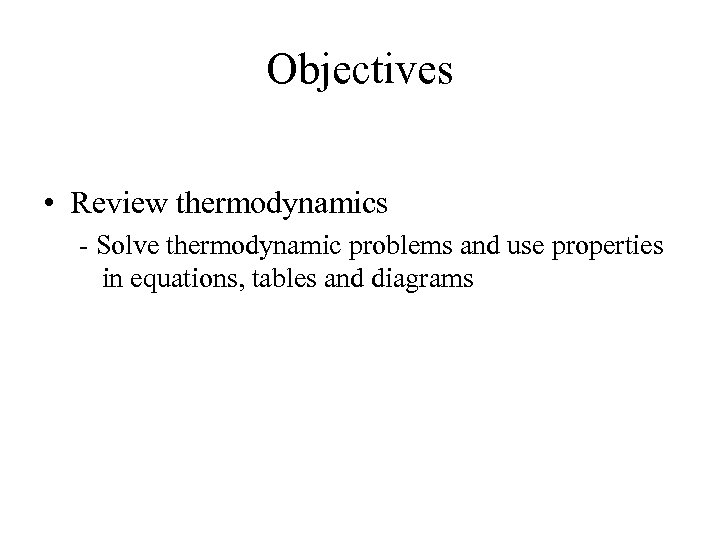Objectives • Review thermodynamics - Solve thermodynamic problems and use properties in equations, tables and diagrams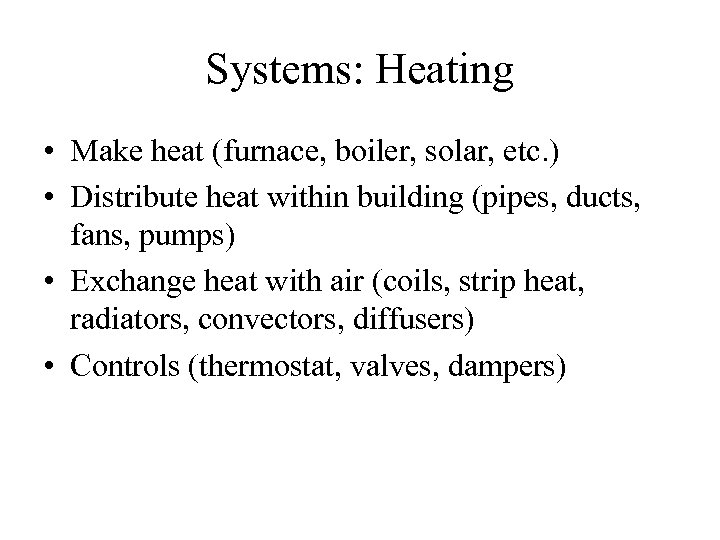Systems: Heating • Make heat (furnace, boiler, solar, etc. ) • Distribute heat within building (pipes, ducts, fans, pumps) • Exchange heat with air (coils, strip heat, radiators, convectors, diffusers) • Controls (thermostat, valves, dampers)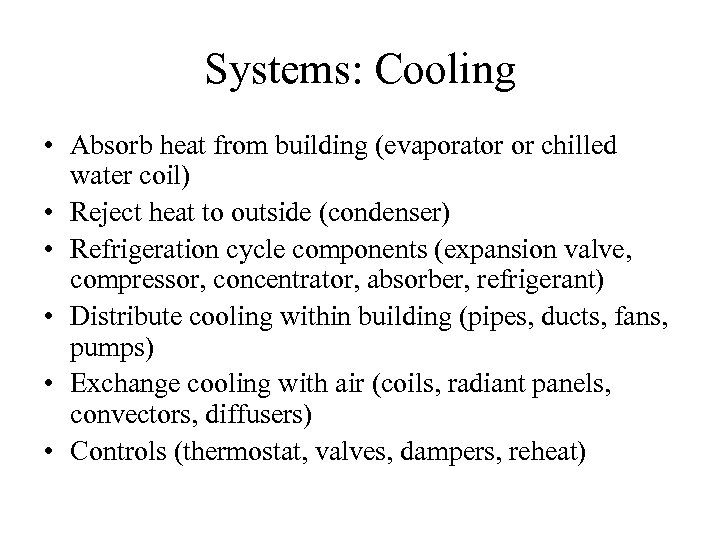Systems: Cooling • Absorb heat from building (evaporator or chilled water coil) • Reject heat to outside (condenser) • Refrigeration cycle components (expansion valve, compressor, concentrator, absorber, refrigerant) • Distribute cooling within building (pipes, ducts, fans, pumps) • Exchange cooling with air (coils, radiant panels, convectors, diffusers) • Controls (thermostat, valves, dampers, reheat)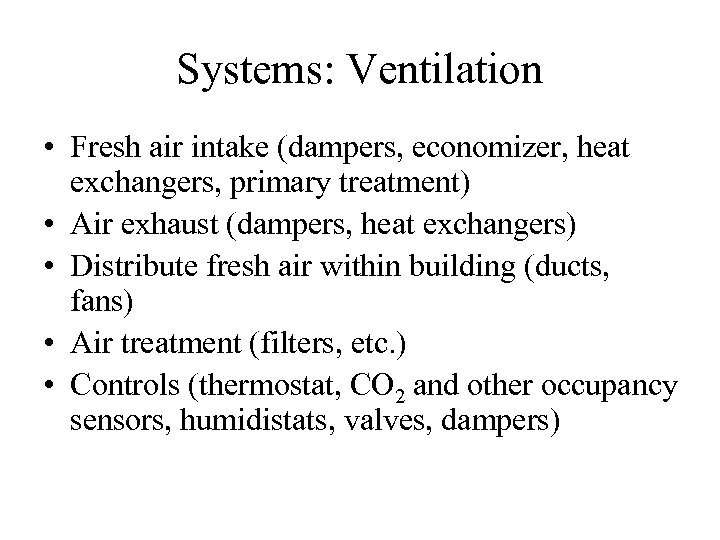Systems: Ventilation • Fresh air intake (dampers, economizer, heat exchangers, primary treatment) • Air exhaust (dampers, heat exchangers) • Distribute fresh air within building (ducts, fans) • Air treatment (filters, etc. ) • Controls (thermostat, CO 2 and other occupancy sensors, humidistats, valves, dampers)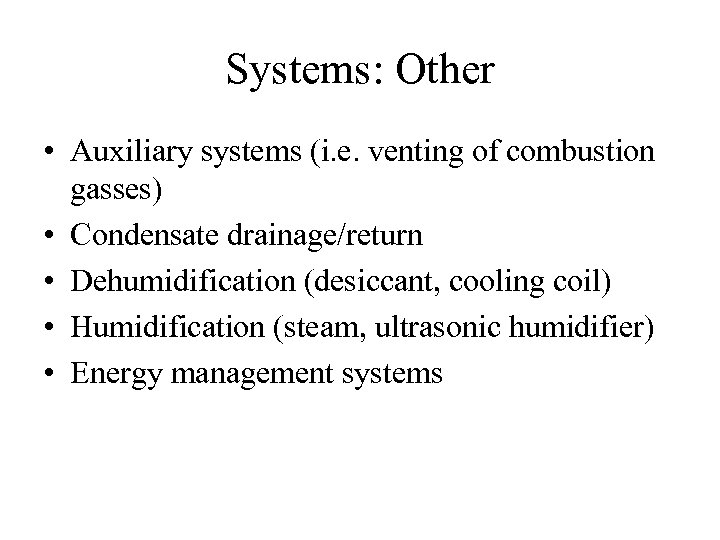Systems: Other • Auxiliary systems (i. e. venting of combustion gasses) • Condensate drainage/return • Dehumidification (desiccant, cooling coil) • Humidification (steam, ultrasonic humidifier) • Energy management systems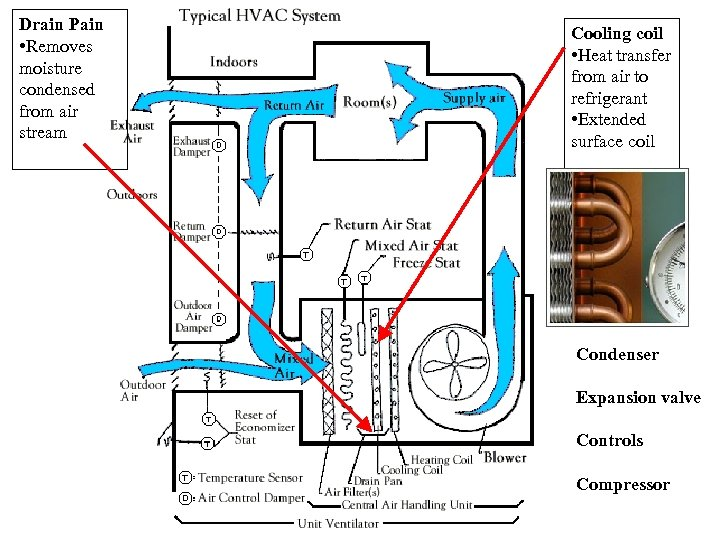Drain Pain • Removes moisture condensed from air stream Cooling coil • Heat transfer from air to refrigerant • Extended surface coil Condenser Expansion valve Controls Compressor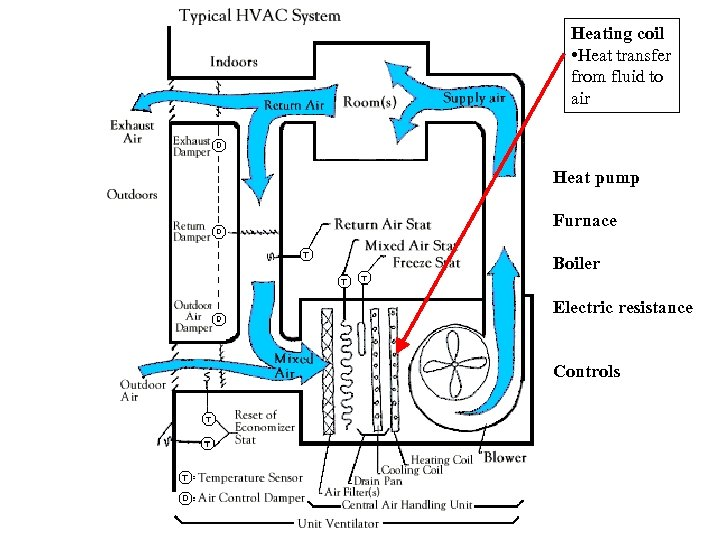Heating coil • Heat transfer from fluid to air Heat pump Furnace Boiler Electric resistance Controls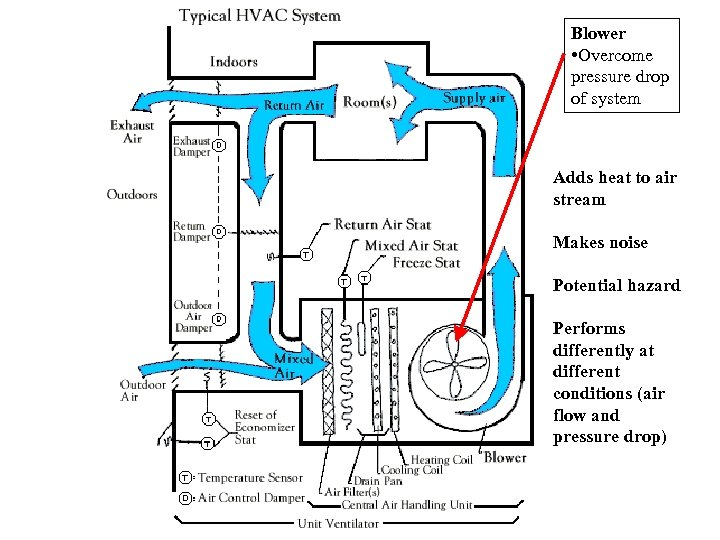Blower • Overcome pressure drop of system Adds heat to air stream Makes noise Potential hazard Performs differently at different conditions (air flow and pressure drop)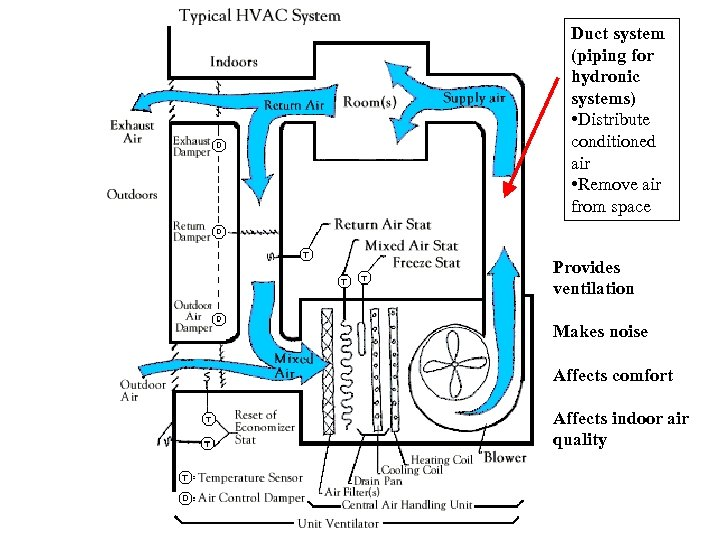Duct system (piping for hydronic systems) • Distribute conditioned air • Remove air from space Provides ventilation Makes noise Affects comfort Affects indoor air quality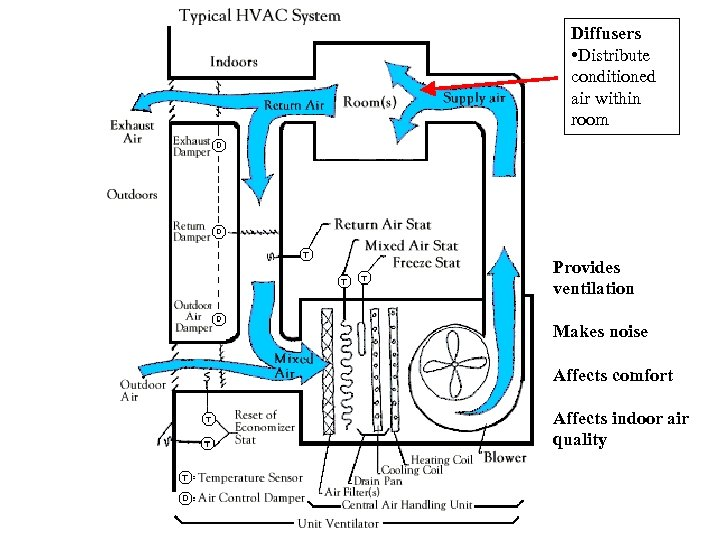Diffusers • Distribute conditioned air within room Provides ventilation Makes noise Affects comfort Affects indoor air quality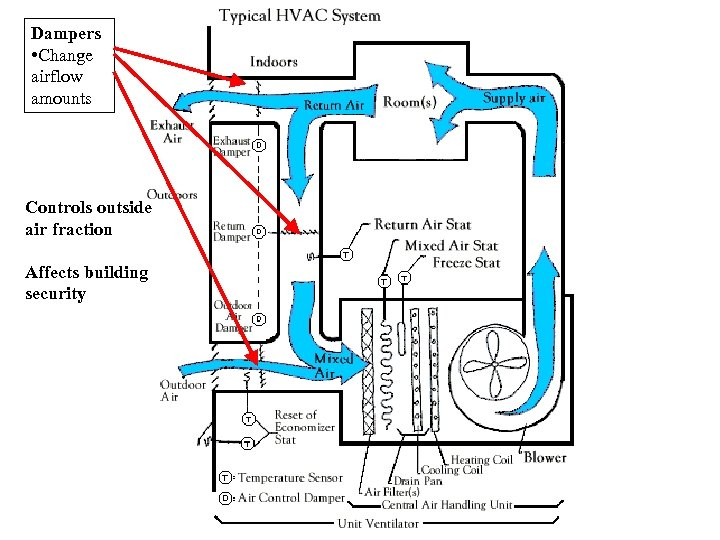Dampers • Change airflow amounts Controls outside air fraction Affects building security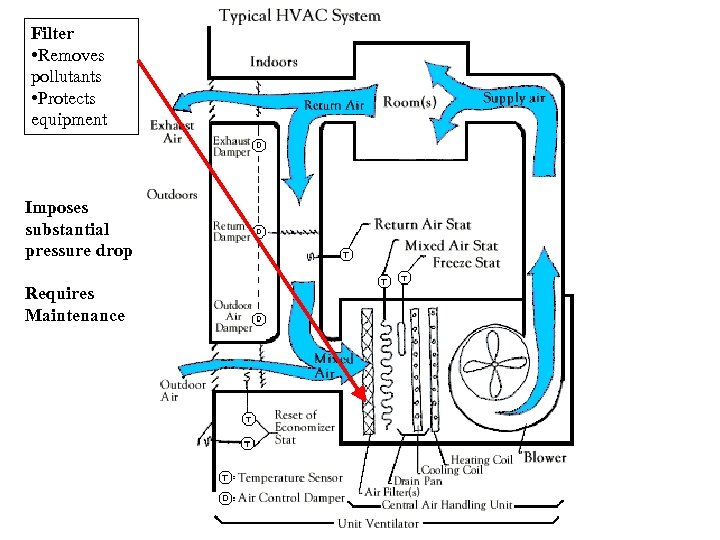Filter • Removes pollutants • Protects equipment Imposes substantial pressure drop Requires Maintenance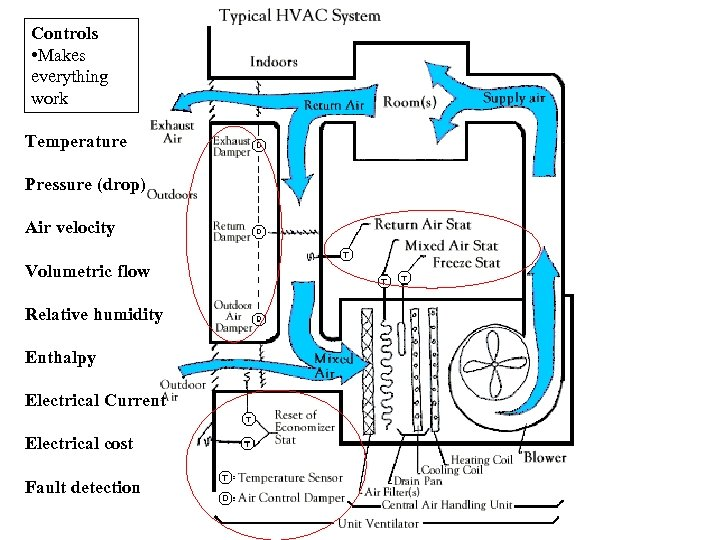Controls • Makes everything work Temperature Pressure (drop) Air velocity Volumetric flow Relative humidity Enthalpy Electrical Current Electrical cost Fault detection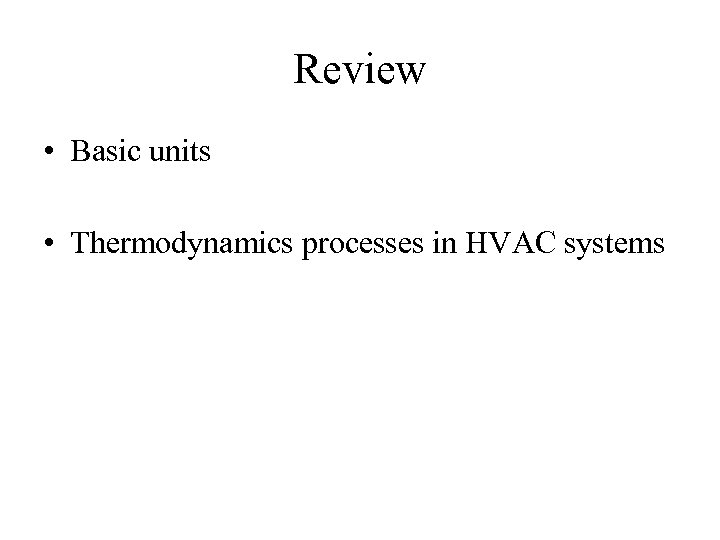Review • Basic units • Thermodynamics processes in HVAC systems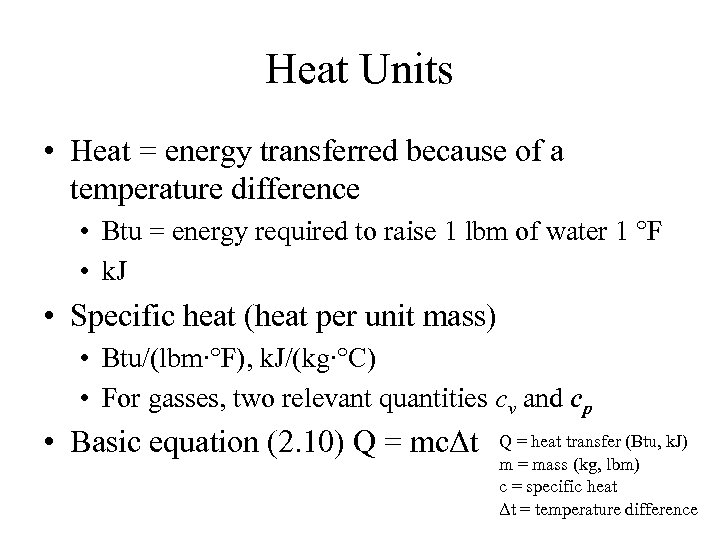Heat Units • Heat = energy transferred because of a temperature difference • Btu = energy required to raise 1 lbm of water 1 °F • k. J • Specific heat (heat per unit mass) • Btu/(lbm∙°F), k. J/(kg∙°C) • For gasses, two relevant quantities cv and cp • Basic equation (2. 10) Q = mcΔt Q = heat transfer (Btu, k. J) m = mass (kg, lbm) c = specific heat Δt = temperature difference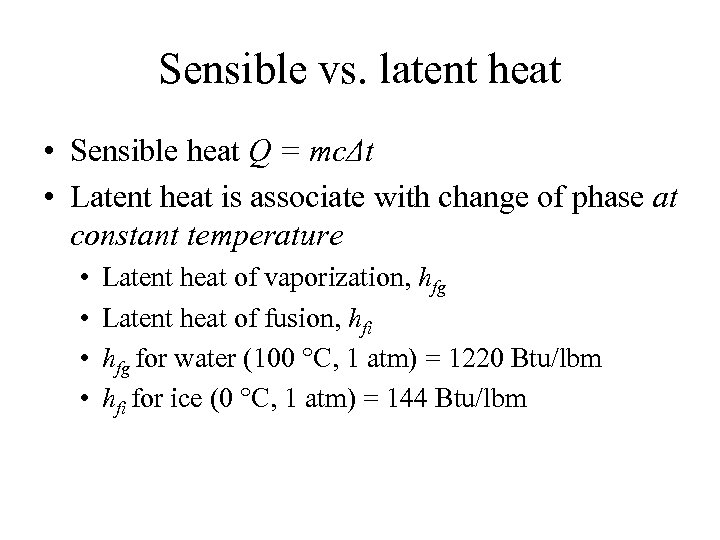Sensible vs. latent heat • Sensible heat Q = mcΔt • Latent heat is associate with change of phase at constant temperature • • Latent heat of vaporization, hfg Latent heat of fusion, hfi hfg for water (100 °C, 1 atm) = 1220 Btu/lbm hfi for ice (0 °C, 1 atm) = 144 Btu/lbm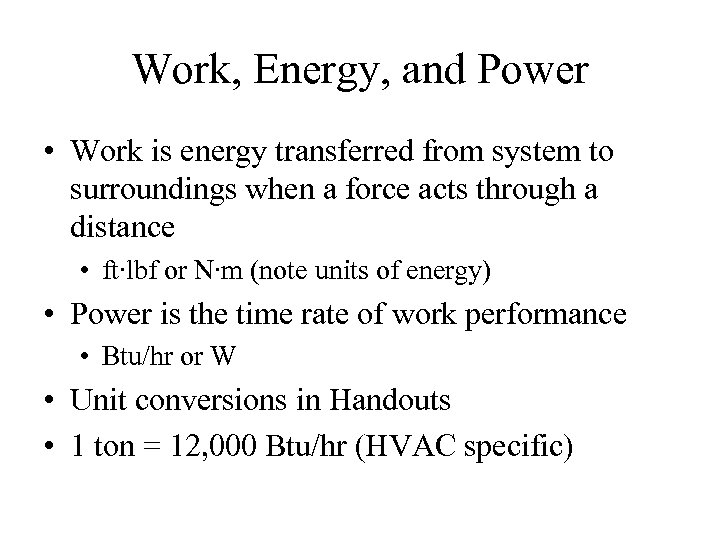Work, Energy, and Power • Work is energy transferred from system to surroundings when a force acts through a distance • ft∙lbf or N∙m (note units of energy) • Power is the time rate of work performance • Btu/hr or W • Unit conversions in Handouts • 1 ton = 12, 000 Btu/hr (HVAC specific)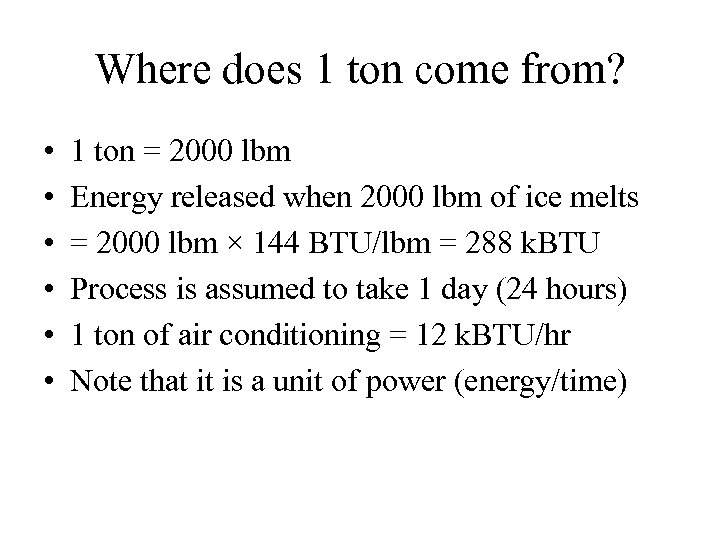Where does 1 ton come from? • • • 1 ton = 2000 lbm Energy released when 2000 lbm of ice melts = 2000 lbm × 144 BTU/lbm = 288 k. BTU Process is assumed to take 1 day (24 hours) 1 ton of air conditioning = 12 k. BTU/hr Note that it is a unit of power (energy/time)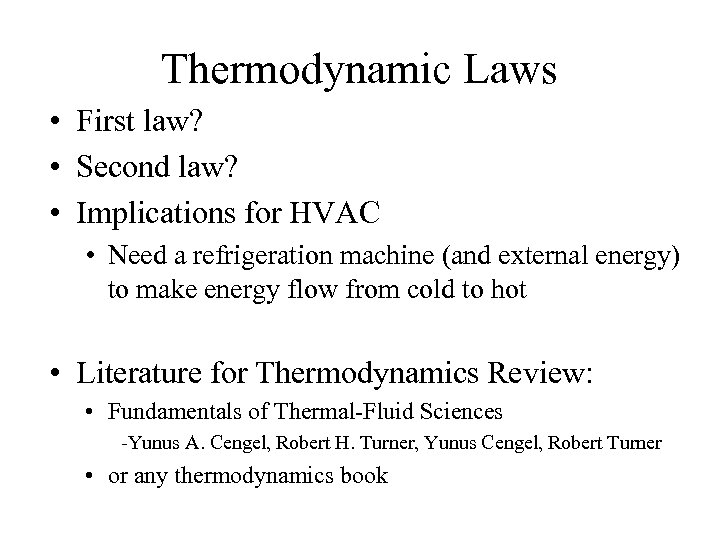Thermodynamic Laws • First law? • Second law? • Implications for HVAC • Need a refrigeration machine (and external energy) to make energy flow from cold to hot • Literature for Thermodynamics Review: • Fundamentals of Thermal-Fluid Sciences -Yunus A. Cengel, Robert H. Turner, Yunus Cengel, Robert Turner • or any thermodynamics book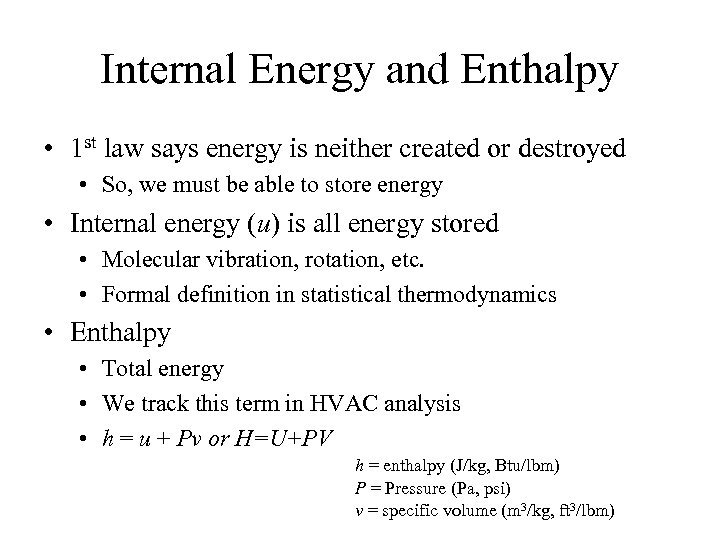Internal Energy and Enthalpy • 1 st law says energy is neither created or destroyed • So, we must be able to store energy • Internal energy (u) is all energy stored • Molecular vibration, rotation, etc. • Formal definition in statistical thermodynamics • Enthalpy • Total energy • We track this term in HVAC analysis • h = u + Pv or H=U+PV h = enthalpy (J/kg, Btu/lbm) P = Pressure (Pa, psi) v = specific volume (m 3/kg, ft 3/lbm)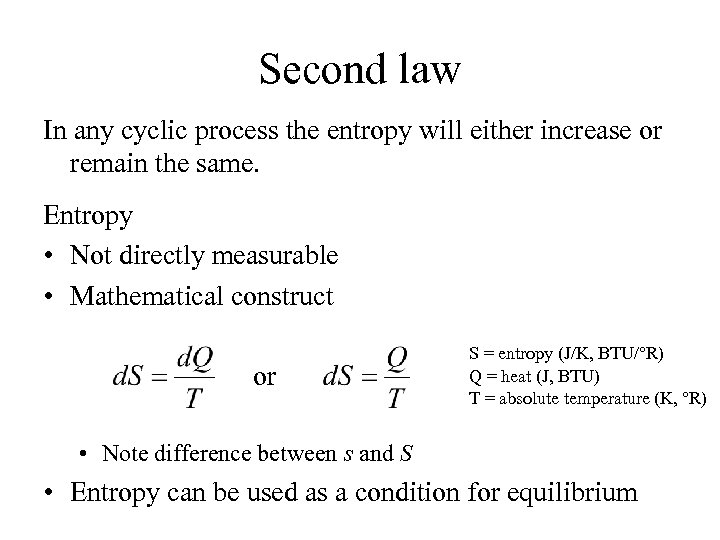Second law In any cyclic process the entropy will either increase or remain the same. Entropy • Not directly measurable • Mathematical construct or S = entropy (J/K, BTU/°R) Q = heat (J, BTU) T = absolute temperature (K, °R) • Note difference between s and S • Entropy can be used as a condition for equilibrium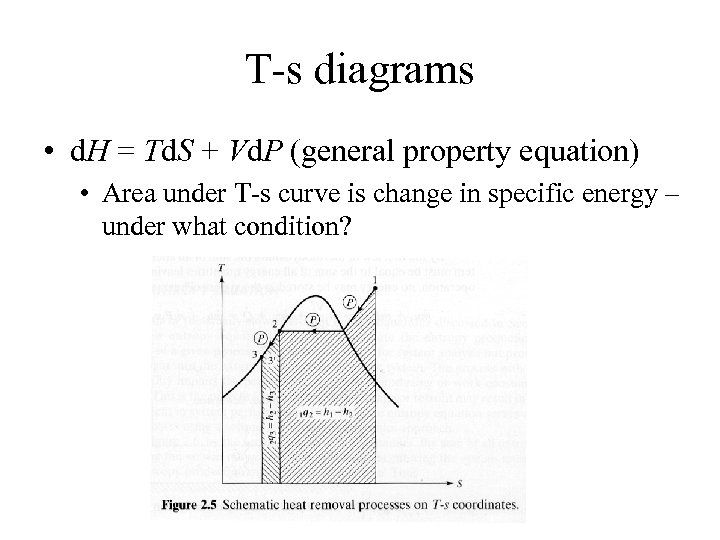T-s diagrams • d. H = Td. S + Vd. P (general property equation) • Area under T-s curve is change in specific energy – under what condition?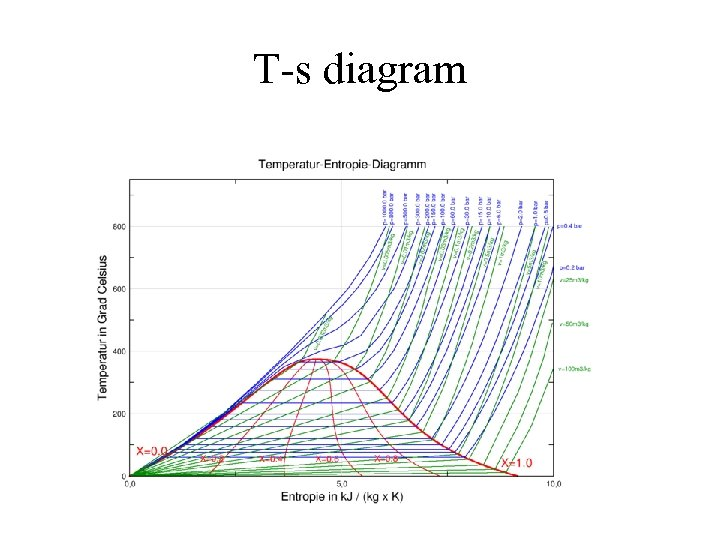T-s diagram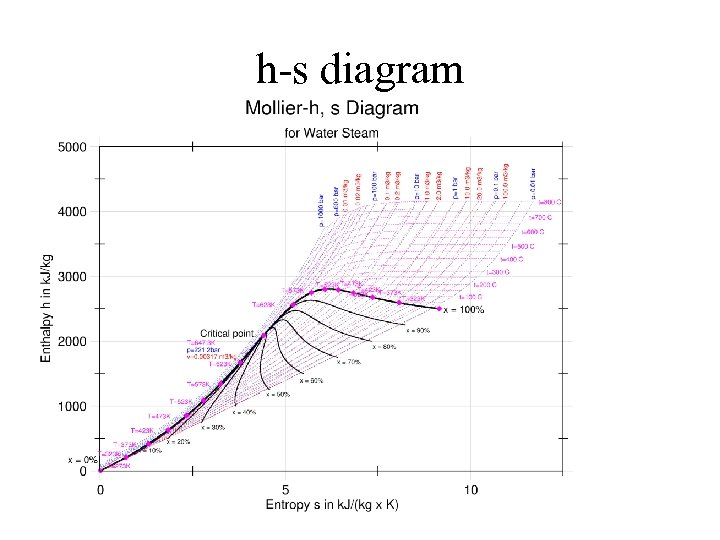h-s diagram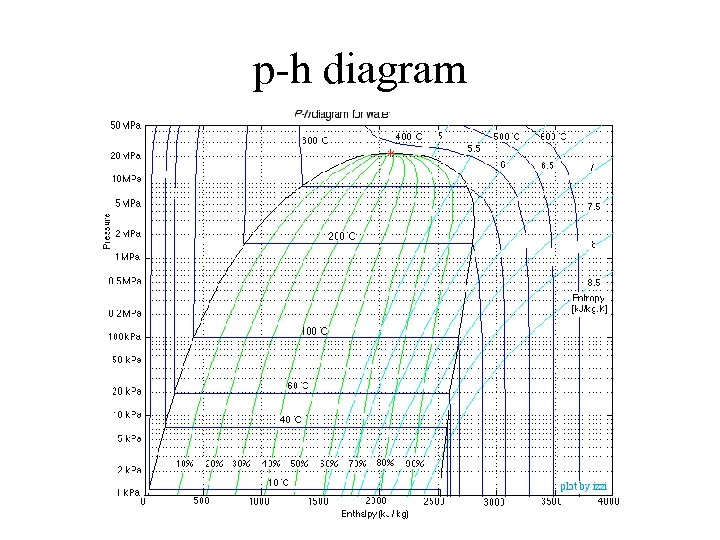p-h diagram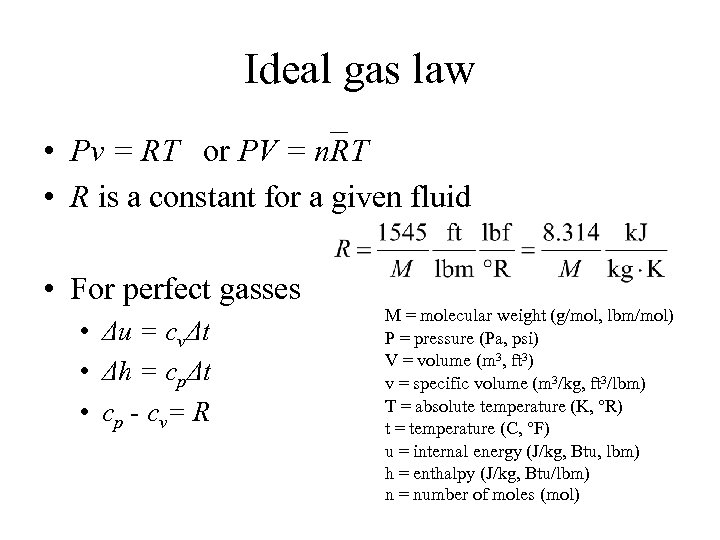Ideal gas law • Pv = RT or PV = n. RT • R is a constant for a given fluid • For perfect gasses • Δu = cvΔt • Δh = cpΔt • c p - c v= R M = molecular weight (g/mol, lbm/mol) P = pressure (Pa, psi) V = volume (m 3, ft 3) v = specific volume (m 3/kg, ft 3/lbm) T = absolute temperature (K, °R) t = temperature (C, °F) u = internal energy (J/kg, Btu, lbm) h = enthalpy (J/kg, Btu/lbm) n = number of moles (mol)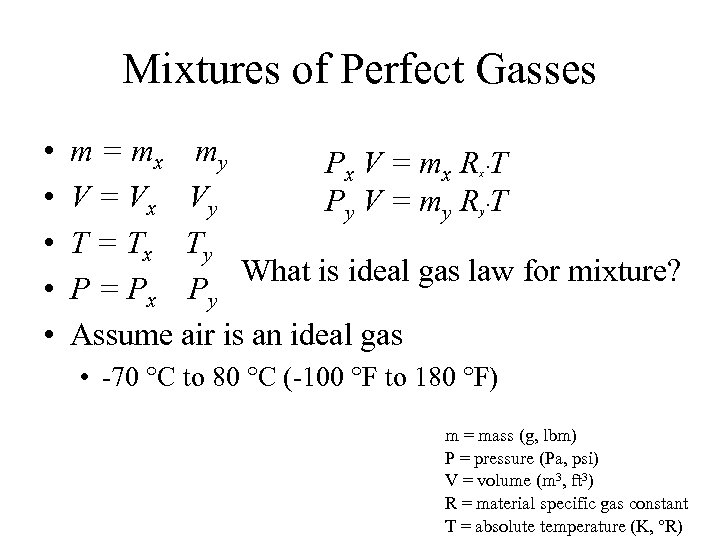Mixtures of Perfect Gasses • • • m = m x my Px V = mx R ∙T V = V x Vy Py V = my R ∙T T = T x Ty What is ideal gas law for mixture? P = P x Py Assume air is an ideal gas x y • -70 °C to 80 °C (-100 °F to 180 °F) m = mass (g, lbm) P = pressure (Pa, psi) V = volume (m 3, ft 3) R = material specific gas constant T = absolute temperature (K, °R)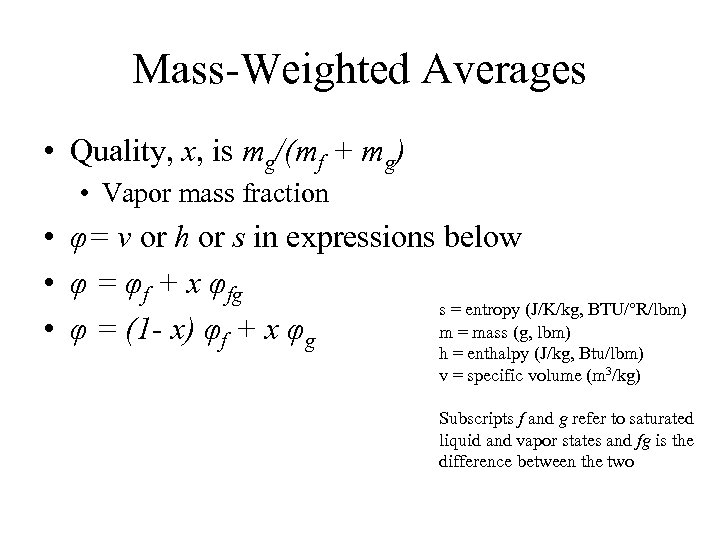Mass-Weighted Averages • Quality, x, is mg/(mf + mg) • Vapor mass fraction • φ= v or h or s in expressions below • φ = φf + x φfg s = entropy (J/K/kg, BTU/°R/lbm) m = mass (g, lbm) • φ = (1 - x) φf + x φg h = enthalpy (J/kg, Btu/lbm) v = specific volume (m 3/kg) Subscripts f and g refer to saturated liquid and vapor states and fg is the difference between the two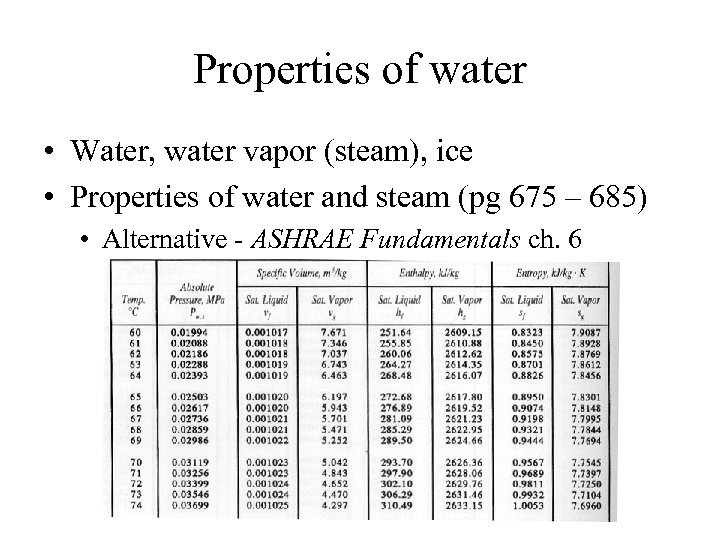Properties of water • Water, water vapor (steam), ice • Properties of water and steam (pg 675 – 685) • Alternative - ASHRAE Fundamentals ch. 6